oomph::Circle Class Reference

//////////////////////////////////////////////////////////////////// More...

#include <geom_objects.h>Inheritance diagram for oomph::Circle:

## Public Member Functions

Circle (const double &x_c, const double &y_c, const double &r)
Constructor: Pass x and y-coords of centre and radius (all pinned) More...

Circle (const double &x_c, const double &y_c, const double &r, TimeStepper *time_stepper_pt)
Constructor: Pass x and y-coords of centre and radius (all pinned) Circle is static but can be used in time-dependent runs with specified timestepper. More...

Circle (const Vector< Data * > &geom_data_pt)
Constructor: Pass x and y-coords of centre and radius (all as Data) More...

Circle (const Circle &dummy)=delete
Broken copy constructor. More...

void operator= (const Circle &)=delete
Broken assignment operator. More...

virtual ~Circle ()
Destructor: Clean up if necessary. More...

void position (const Vector< double > &zeta, Vector< double > &r) const
Position Vector at Lagrangian coordinate zeta. More...

void position (const unsigned &t, const Vector< double > &zeta, Vector< double > &r) const
Parametrised position on object: r(zeta). Evaluated at previous timestep. t=0: current time; t>0: previous timestep. More...

double & x_c ()
Access function to x-coordinate of centre of circle. More...

double & y_c ()
Access function to y-coordinate of centre of circle. More...

double & R ()
Access function to radius of circle. More...

unsigned ngeom_data () const
How many items of Data does the shape of the object depend on? More...

Datageom_data_pt (const unsigned &j)
Return pointer to the j-th Data item that the object's shape depends on. More...Public Member Functions inherited from oomph::GeomObject
GeomObject ()
Default constructor. More...

GeomObject (const unsigned &ndim)
Constructor: Pass dimension of geometric object (# of Eulerian coords = # of Lagrangian coords; no time history available/needed) More...

GeomObject (const unsigned &nlagrangian, const unsigned &ndim)
Constructor: pass # of Eulerian and Lagrangian coordinates. No time history available/needed. More...

GeomObject (const unsigned &nlagrangian, const unsigned &ndim, TimeStepper *time_stepper_pt)
Constructor: pass # of Eulerian and Lagrangian coordinates and pointer to time-stepper which is used to handle the position at previous timesteps and allows the evaluation of veloc/acceleration etc. in cases where the GeomData varies with time. More...

GeomObject (const GeomObject &dummy)=delete
Broken copy constructor. More...

void operator= (const GeomObject &)=delete
Broken assignment operator. More...

virtual ~GeomObject ()
(Empty) destructor More...

unsigned nlagrangian () const
Access function to # of Lagrangian coordinates. More...

unsigned ndim () const
Access function to # of Eulerian coordinates. More...

void set_nlagrangian_and_ndim (const unsigned &n_lagrangian, const unsigned &n_dim)
Set # of Lagrangian and Eulerian coordinates. More...

TimeStepper *& time_stepper_pt ()
Access function for pointer to time stepper: Null if object is not time-dependent. More...

TimeSteppertime_stepper_pt () const
Access function for pointer to time stepper: Null if object is not time-dependent. Const version. More...

virtual void position (const double &t, const Vector< double > &zeta, Vector< double > &r) const
Parametrised position on object: r(zeta). Evaluated at the continuous time value, t. More...

virtual void dposition_dt (const Vector< double > &zeta, const unsigned &j, Vector< double > &drdt)
j-th time-derivative on object at current time: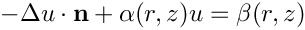. More...

virtual void dposition (const Vector< double > &zeta, DenseMatrix< double > &drdzeta) const
Derivative of position Vector w.r.t. to coordinates: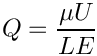= drdzeta(alpha,i). Evaluated at current time. More...

virtual void d2position (const Vector< double > &zeta, RankThreeTensor< double > &ddrdzeta) const
2nd derivative of position Vector w.r.t. to coordinates: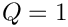= ddrdzeta(alpha,beta,i). Evaluated at current time. More...

virtual void d2position (const Vector< double > &zeta, Vector< double > &r, DenseMatrix< double > &drdzeta, RankThreeTensor< double > &ddrdzeta) const
Posn Vector and its 1st & 2nd derivatives w.r.t. to coordinates:= drdzeta(alpha,i).= ddrdzeta(alpha,beta,i). Evaluated at current time. More...

virtual void locate_zeta (const Vector< double > &zeta, GeomObject *&sub_geom_object_pt, Vector< double > &s, const bool &use_coordinate_as_initial_guess=false)
A geometric object may be composed of may sub-objects (e.g. a finite-element representation of a boundary). In order to implement sparse update functions, it is necessary to know the sub-object and local coordinate within that sub-object at a given intrinsic coordinate, zeta. Note that only one sub-object can "cover" any given intrinsic position. If the position is at an "interface" between sub-objects, either one can be returned. The default implementation merely returns, the pointer to the "entire" GeomObject and the coordinate, zeta The optional boolean flag only applies if a Newton method is used to find the value of zeta, and if true the value of the coordinate s is used as the initial guess for the method. If the flag is false (the default) a value of s=0 is used as the initial guess. More...

virtual void interpolated_zeta (const Vector< double > &s, Vector< double > &zeta) const
A geometric object may be composed of many sub-objects each with their own local coordinate. This function returns the "global" intrinsic coordinate zeta (within the compound object), at a given local coordinate s (i.e. the intrinsic coordinate of the sub-GeomObject. In simple (non-compound) GeomObjects, the local intrinsic coordinate is the global intrinsic coordinate and so the function merely returns s. To make it less likely that the default implementation is called in error (because it is not overloaded in a derived GeomObject where the default is not appropriate, we do at least check that s and zeta have the same size if called in PARANOID mode. More...

## Protected Attributes

Vector< Data * > Geom_data_pt
Vector of pointers to Data items that affects the object's shape. More...

bool Must_clean_up
Do I need to clean up? More...

bool Is_time_dependent
Genuine time-dependence? More...Protected Attributes inherited from oomph::GeomObject
unsigned NLagrangian
Number of Lagrangian (intrinsic) coordinates. More...

unsigned Ndim
Number of Eulerian coordinates. More...

TimeStepperGeom_object_time_stepper_pt

## Detailed Description

////////////////////////////////////////////////////////////////////

//////////////////////////////////////////////////////////////////// Circle in 2D space.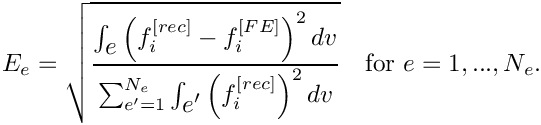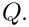Definition at line 872 of file geom_objects.h.

## ◆ Circle() [1/4]

 oomph::Circle::Circle ( const double & x_c, const double & y_c, const double & r )
inline

Constructor: Pass x and y-coords of centre and radius (all pinned)

Definition at line 876 of file geom_objects.h.

References Geom_data_pt, Is_time_dependent, Must_clean_up, x_c(), and y_c().

## ◆ Circle() [2/4]

 oomph::Circle::Circle ( const double & x_c, const double & y_c, const double & r, TimeStepper * time_stepper_pt )
inline

Constructor: Pass x and y-coords of centre and radius (all pinned) Circle is static but can be used in time-dependent runs with specified timestepper.

Definition at line 915 of file geom_objects.h.

## ◆ Circle() [3/4]

 oomph::Circle::Circle ( const Vector< Data * > & geom_data_pt )
inline

Constructor: Pass x and y-coords of centre and radius (all as Data)

Geom_data_pt->value(0) = X_c;
Geom_data_pt->value(1) = Y_c;
Geom_data_pt->value(2) = R;
Vector< Data * > Geom_data_pt
Vector of pointers to Data items that affects the object's shape.
double & R()
Access function to radius of circle.

Definition at line 964 of file geom_objects.h.

## ◆ Circle() [4/4]

 oomph::Circle::Circle ( const Circle & dummy )
delete

Broken copy constructor.

## ◆ ~Circle()

 virtual oomph::Circle::~Circle ( )
inlinevirtual

Destructor: Clean up if necessary.

Definition at line 1009 of file geom_objects.h.

References Geom_data_pt, i, Must_clean_up, and ngeom_data().

## ◆ geom_data_pt()

 Data * oomph::Circle::geom_data_pt ( const unsigned & j )
inlinevirtual

Return pointer to the j-th Data item that the object's shape depends on.

Reimplemented from oomph::GeomObject.

Definition at line 1102 of file geom_objects.h.

References Geom_data_pt.

Referenced by Circle().

## ◆ ngeom_data()

 unsigned oomph::Circle::ngeom_data ( ) const
inlinevirtual

How many items of Data does the shape of the object depend on?

Reimplemented from oomph::GeomObject.

Definition at line 1095 of file geom_objects.h.

References Geom_data_pt.

Referenced by ~Circle().

## ◆ operator=()

 void oomph::Circle::operator= ( const Circle & )
delete

Broken assignment operator.

## ◆ position() [1/2]

 void oomph::Circle::position ( const unsigned & t, const Vector< double > & zeta, Vector< double > & r ) const
inlinevirtual

Parametrised position on object: r(zeta). Evaluated at previous timestep. t=0: current time; t>0: previous timestep.

Reimplemented from oomph::GeomObject.

Definition at line 1040 of file geom_objects.h.

References Geom_data_pt, Is_time_dependent, position(), R(), t, and oomph::GeomObject::time_stepper_pt().

## ◆ position() [2/2]

 void oomph::Circle::position ( const Vector< double > & zeta, Vector< double > & r ) const
inlinevirtual

Position Vector at Lagrangian coordinate zeta.

Implements oomph::GeomObject.

Definition at line 1024 of file geom_objects.h.

References Geom_data_pt, and R().

Referenced by oomph::CylinderWithFlagDomain::macro_element_boundary(), and position().

## ◆ R()

 double & oomph::Circle::R ( )
inline

Access function to radius of circle.

Definition at line 1089 of file geom_objects.h.

References Geom_data_pt.

Referenced by position().

## ◆ x_c()

 double & oomph::Circle::x_c ( )
inline

Access function to x-coordinate of centre of circle.

Definition at line 1077 of file geom_objects.h.

References Geom_data_pt.

Referenced by Circle().

## ◆ y_c()

 double & oomph::Circle::y_c ( )
inline

Access function to y-coordinate of centre of circle.

Definition at line 1083 of file geom_objects.h.

References Geom_data_pt.

Referenced by Circle().

## ◆ Geom_data_pt

 Vector oomph::Circle::Geom_data_pt
protected

Vector of pointers to Data items that affects the object's shape.

Definition at line 1109 of file geom_objects.h.

Referenced by Circle(), geom_data_pt(), ngeom_data(), position(), R(), x_c(), y_c(), and ~Circle().

## ◆ Is_time_dependent

 bool oomph::Circle::Is_time_dependent
protected

Genuine time-dependence?

Definition at line 1115 of file geom_objects.h.

Referenced by Circle(), and position().

## ◆ Must_clean_up

 bool oomph::Circle::Must_clean_up
protected

Do I need to clean up?

Definition at line 1112 of file geom_objects.h.

Referenced by Circle(), and ~Circle().

The documentation for this class was generated from the following file: